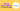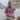# String Interpolation in JavaScript

Updated July 31, 2020Start discussion

String interpolation is replacing placeholders with values in a string literal.

The string interpolation in JavaScript is performed by template literals (strings wrapped in backticks ```) and `\${expression}` as a placeholder. For example:

``````const number = 42;
const message = `The number is \${number}`;

message; // => 'The number is 42'``````

Let’s see in more detail, with examples and best practices, how to use template strings to perform string interpolation in JavaScript.

## 1. The string literals

In JavaScript, there are 3 ways to create string literals.

First, which I prefer for plain strings, is to wrap the string into a pair of single quotes `'`:

``const message = 'Hello, World!';``

The second, which I use rarely, is to wrap the string into a pair of double quotes `"`:

``const message = "Hello, World";``

The third, which permits string interpolation, is to wrap the string into a pair of backticks ```:

``const message = `Hello, World!`;``

The string literal wrapped in backticks ``` is also named template string. This is the literal that supports the string interpolation.

## 2. The placeholders

The template string supports placeholders. The expression inside the placeholder is evaluated during runtime, and the result is inserted into the string.

The placeholder has a special format: `\${expressionToEvaluate}`. The expression inside the placeholder can be of any kind:

• variables: `\${myVar}`
• operators: `\${n1 + n2}`, `\${cond ? 'val 1' : 'val 2'}`
• even function calls `\${myFunc('argument')}`

Here’s an example:

``````const greeting = 'Hello';
const who = 'World';

const message = `\${greeting}, \${who}!`;message; // => 'Hello, World!'``````

``\${greeting}, \${who}!`` is a template string having placeholders `\${greeting}` and `\${who}`.

On script execution, the first placeholder `\${greeting}` is replaced with the value of `greeting` variable, and the same for `\${who}`. The string interpolation result is `'Hello, World!'`.

You can put any expression inside the placeholder: either an operator, a function call, or even more complex expressions.

``````const n1 = 2;
const n2 = 3;

const message1 = `The sum is \${n1 + n2}`;message1; // => 'The sum is 5';

function sum(num1, num2) {
return num1 + num2;
}
const message2 = `The sum is \${sum(n1, n2)}`;message2; // => 'The sum is 5'``````

`\${n1 + n2}` is a placeholder consisting of the addition operator and 2 operands.

While the placeholder `\${sum(n1, n2)}` contains a function invocation.

### 2.1 Implicit to string conversion

The placeholder expression result is implicitly converted to a string.

For example, a number in a placeholder is transformed into a string:

``````const n = 3.5;
const message = `The number is \${n}`;

message; // => `The number is 3.5```````

The expression `n` of the placeholder `\${n}` is evaluated to number `3.5`. The number `3.5` is then transformed into a string `'3.5'`, and inserted into the interpolation result: `'The number is 3.5'`.

If the placeholder contains an object, following the conversion to string rule, the object is converted to a string too. The `toString()` method of the object is called to get the string representation of the object.

For example, let’s insert an array into a template string:

``````const numbers = [1, 2, 3];
const message = `The numbers are \${numbers}`;

message; // => 'The numbers are 1,2,3'``````

The placeholder `\${numbers}` contains an array of numbers.

`toString()` array method executes `array.join(',')` when the array is converted to string. Thus the string interpolation result is `'The numbers are 1,2,3'`.

## 3. Escaping placeholders

Because the placeholder format `\${expression}` has a special meaning in the template literals, you cannot use the sequence of characters `"\${someCharacters}"` without escaping.

For example, let’s try to create a string literal containing the sequence of characters `\${abc}`:

``````const message = `Some weird characters: \${abc}`;
// Throws "ReferenceError: abc is not defined"``````

Inserting `\${abc}` directly throws an error because JavaScript interprets `\${abc}` as a placeholder.

A backslash `\` before the placeholder-like sequence of characters `\\${abc}` solves the problem:

``````const message = `Some weird characters: \\${abc}`;
message; // => 'Some weird characters follow: \${abc}'``````

In the template string ``Some weird characters: \\${abc}`` JavaScript interprets `\\${abc}` as a sequence of characters, rather than a placeholder.

Alongside with `\${abc}`, the sequence of characters like `\${abc` and `\${` also have to be escaped with a backslash `\`:

``````const message = `Some weird characters: \\${abc} \\${abc \\${`;

message; // => 'Some weird characters: \${abc} \${abc \${'``````

## 4. Best practices

### 4.1 Refactor string concatentation

The string interpolation should be used instead of string concatenation to construct lengthy strings.

If for some reason you’re still concatenating string literals and expressions using `+` operator:

``````const n1 = 2;
const n2 = 3;
const message = 'The sum of ' + n1 + ' and ' + n2 + ' is ' + (n1 + n2);
message; // => 'The sum of 2 and 3 is 5'``````

Then it’s the time to switch to string interpolation using template strings:

``````const n1 = 2;
const n2 = 3;
const message = `The sum of \${n1} and \${n2} is \${n1 + n2}`;
message; // => 'The sum of 2 and 3 is 5'``````

The template string usage requires less code and is easier to read.

### 4.2 Helper variables

When the template string contains many complex expressions, it might decrease the readability of the literal.

Here’s a template string having placeholders with complex expressions:

``````const n1 = 2;
const n2 = 3;

const message =
`Sum: \${n1 + n2}, difference: \${n1 - n2}, pow: \${Math.pow(n1, n2)}`;

message; // => 'Sum: 5, difference: -1, pow: 8'``````

The more complex the placeholders are, the more tempting is to add helper variables to store intermediate values.

``````const n1 = 2;
const n2 = 3;

const sum = n1 + n2;const diff = n1 - n2;const pow = Math.pow(n1, n2);
const message = `Sum: \${sum}, difference: \${diff}, pow: \${pow}`;

message; // => 'Sum: 5, difference: -1, pow: 8'``````

With the introduction of helper variables `sum`, `diff`, and `pow`, the template string becomes lighter. Additionally, the code self-documents when the intermediate variables are used.

### 4.3 Single quotes in placeholders

I recommended using single quotes `'` rather than backticks ``` in the expressions inside the placeholder.

Let’s use the ternary operator. When the placeholder uses backticks ``` it’s quite difficult to grasp the template string because there are too many backticks in the template string:

``````function getLoadingMessage(isLoading) {

But using single quotes inside the placeholder just looks better:

``````function getLoadingMessage(isLoading) {

### 4.4 Alternative solutions

The string interpolation is helpful in many situations. But when the template string becomes large, with complex placeholder expressions, you might look for other solutions.

The following component builds the CSS class based on 2 variables:

``````function LoadingMessage({ isLoading, isModal }) {
const className =
return (
<div className={className}>
</div>
);
}``````

The template literal that determines the class name has 2 ternary operators and a mix of string literals. You may find it difficult to understand.

A tool like classnames might be a better choice than template string. The tool constructs the class name in a more declarative way.

Let’s refactor the component to use `classnames`:

``````import classNames from 'classnames';

return (
<div className={className}>
</div>
);
}``````

This version of the component, which uses the `classnames` tool, is easier to understand than the template string version.

Adding more CSS classes (for example to handle `isErrorLoading`) would not decrease the readability of the version that uses `classnames` utility (contrary to using a template literal).

## 5. String interpolation in TypeScript

The string interpolation in TypeScript works the same way as in JavaScript:

``````const count: number = 10;
const message: string = `You have \${count} products`;

message; // => 'You have 10 products'``````

## 6. String interpolation in React

The string interpolation in React consists of 2 cases:

• Attribute interpolation: `attribute={`My string \${placeholder}`}`
• Element’s text interpolation: `<span>My string {placeholder}</span>`

Here’s an example that shows both cases:

``````import React from 'react';

return (
<button
>
);
}``````

`<BuyProductsButton count={10} buy={() => {}} />` would render the output:

``````<button title="Buy 10 products">
</button>``````

During the interpolation of both attribute and text strings, placeholders `\${count}` and correspondingly `{count}` are replaced with `10`.

## 7. Conclusion

The string interpolation is a great feature because it helps to insert values into string literals in a concise and readable manner. And avoid the clumsy string concatenation approach.

In JavaScript, the template string implements the string interpolation.

A template string is defined by wrapping a sequence of characters into a pair of backticks ``I'm template string``. The template string placeholders have the format `\${expression}`, for example ``The number is \${number}``.

Don’t overcomplicate the string literal. If the template string uses complex expressions, try to introduce intermediate variables to store the expressions before putting them into placeholders.

As soon as you need a value inserted into a string literal, the template string is the way to go.

## Quality posts into your inbox

I regularly publish posts containing:

• Important JavaScript concepts explained in simple words
• Overview of new JavaScript features
• How to use TypeScript and typing
• Software design and good coding practices